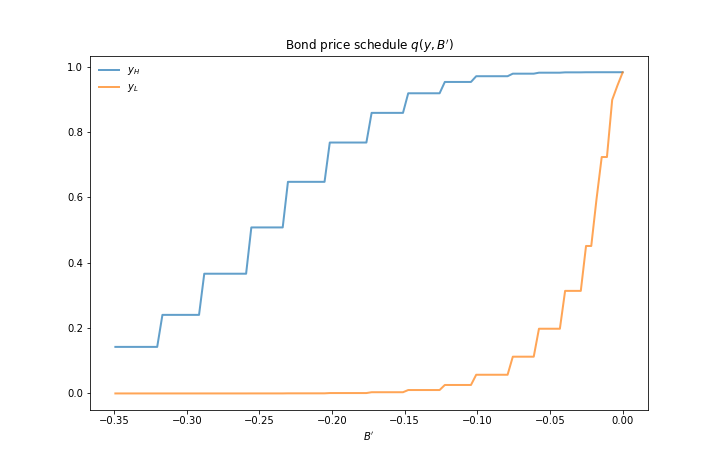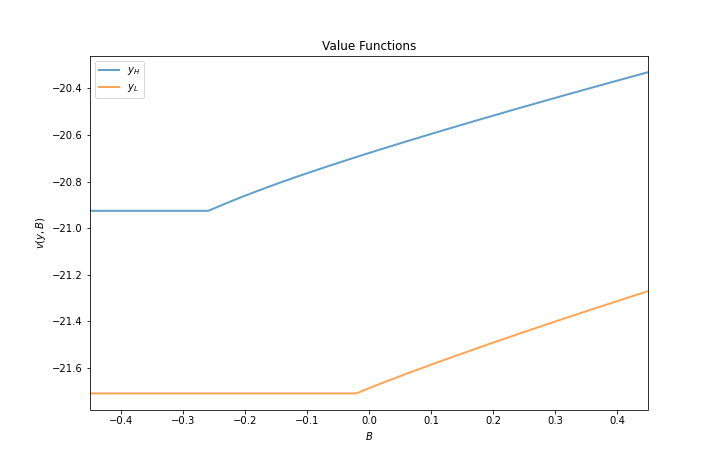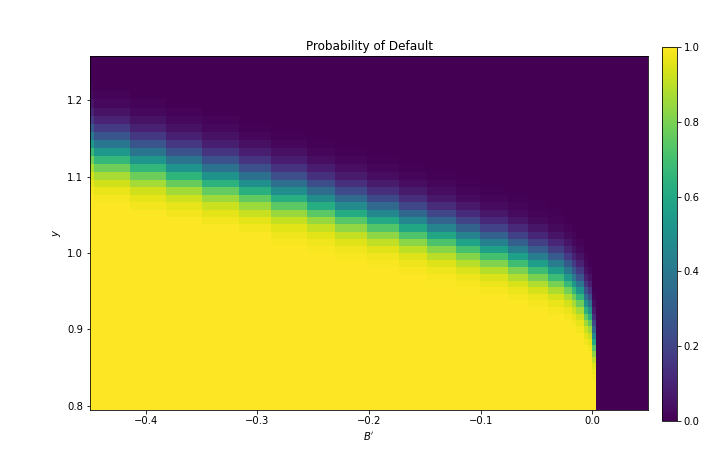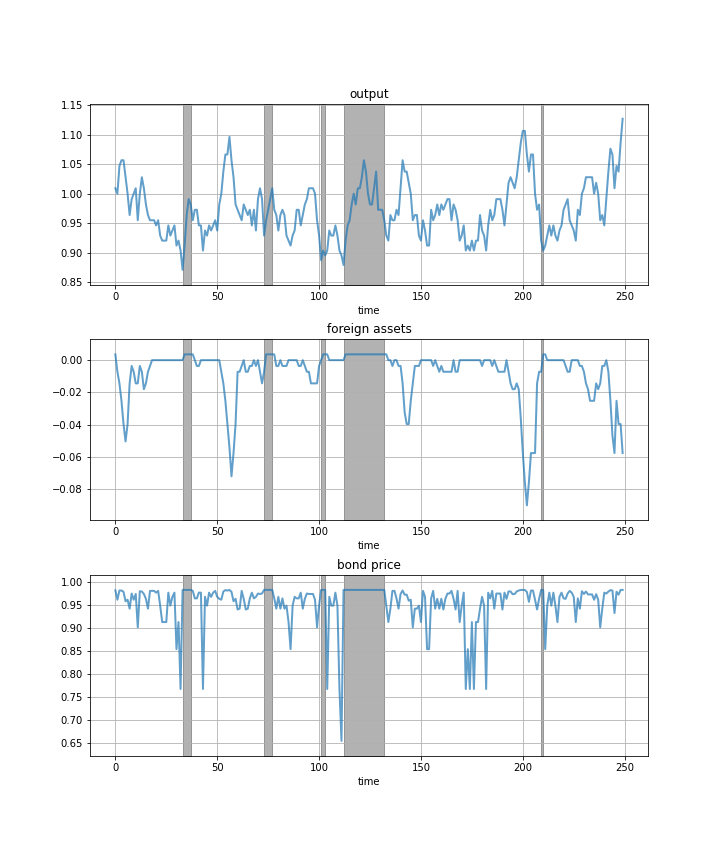# Default Risk and Income Fluctuations¶

## Contents¶

In addition to what’s in Anaconda, this lecture will need the following libraries:

In [ ]:
!pip install --upgrade quantecon


## Overview¶

This lecture computes versions of Arellano’s [Are08] model of sovereign default.

The model describes interactions among default risk, output, and an equilibrium interest rate that includes a premium for endogenous default risk.

The decision maker is a government of a small open economy that borrows from risk-neutral foreign creditors.

The foreign lenders must be compensated for default risk.

The government borrows and lends abroad in order to smooth the consumption of its citizens.

The government repays its debt only if it wants to, but declining to pay has adverse consequences.

The interest rate on government debt adjusts in response to the state-dependent default probability chosen by government.

The model yields outcomes that help interpret sovereign default experiences, including

• countercyclical interest rates on sovereign debt
• high volatility of consumption relative to output

Notably, long recessions caused by bad draws in the income process increase the government’s incentive to default.

• spikes in interest rates
• large drops in output, consumption, and welfare
• large capital outflows during recessions

Such dynamics are consistent with experiences of many countries.

In [ ]:
import matplotlib.pyplot as plt
import numpy as np
import quantecon as qe
import random

from numba import jit, int64, float64
from numba.experimental import jitclass
%matplotlib inline


## Structure¶

In this section we describe the main features of the model.

### Output, Consumption and Debt¶

A small open economy is endowed with an exogenous stochastically fluctuating potential output stream $\{y_t\}$.

Potential output is realized only in periods in which the government honors its sovereign debt.

The output good can be traded or consumed.

The sequence $\{y_t\}$ is described by a Markov process with stochastic density kernel $p(y, y')$.

Households within the country are identical and rank stochastic consumption streams according to

$$\mathbb E \sum_{t=0}^{\infty} \beta^t u(c_t) \tag{15.1}$$

Here

• $0 < \beta < 1$ is a time discount factor
• $u$ is an increasing and strictly concave utility function

Consumption sequences enjoyed by households are affected by the government’s decision to borrow or lend internationally.

The government is benevolent in the sense that its aim is to maximize (15.1).

The government is the only domestic actor with access to foreign credit.

Because household are averse to consumption fluctuations, the government will try to smooth consumption by borrowing from (and lending to) foreign creditors.

### Asset Markets¶

The only credit instrument available to the government is a one-period bond traded in international credit markets.

The bond market has the following features

• The bond matures in one period and is not state contingent.
• A purchase of a bond with face value $B'$ is a claim to $B'$ units of the consumption good next period.
• To purchase $B'$ next period costs $q B'$ now, or, what is equivalent.
• For selling $-B'$ units of next period goods the seller earns $- q B'$ of today’s goods.
• If $B' < 0$, then $-q B'$ units of the good are received in the current period, for a promise to repay $-B'$ units next period.
• There is an equilibrium price function $q(B', y)$ that makes $q$ depend on both $B'$ and $y$.

Earnings on the government portfolio are distributed (or, if negative, taxed) lump sum to households.

When the government is not excluded from financial markets, the one-period national budget constraint is

$$c = y + B - q(B', y) B' \tag{15.2}$$

Here and below, a prime denotes a next period value or a claim maturing next period.

To rule out Ponzi schemes, we also require that $B \geq -Z$ in every period.

• $Z$ is chosen to be sufficiently large that the constraint never binds in equilibrium.

### Financial Markets¶

Foreign creditors

• are risk neutral
• know the domestic output stochastic process $\{y_t\}$ and observe $y_t, y_{t-1}, \ldots,$ at time $t$
• can borrow or lend without limit in an international credit market at a constant international interest rate $r$
• receive full payment if the government chooses to pay
• receive zero if the government defaults on its one-period debt due

When a government is expected to default next period with probability $\delta$, the expected value of a promise to pay one unit of consumption next period is $1 - \delta$.

Therefore, the discounted expected value of a promise to pay $B$ next period is

$$q = \frac{1 - \delta}{1 + r} \tag{15.3}$$

Next we turn to how the government in effect chooses the default probability $\delta$.

### Government’s Decisions¶

At each point in time $t$, the government chooses between

1. defaulting
2. meeting its current obligations and purchasing or selling an optimal quantity of one-period sovereign debt

Defaulting means declining to repay all of its current obligations.

If the government defaults in the current period, then consumption equals current output.

But a sovereign default has two consequences:

1. Output immediately falls from $y$ to $h(y)$, where $0 \leq h(y) \leq y$.
• It returns to $y$ only after the country regains access to international credit markets.

### Reentering International Credit Market¶

While in a state of default, the economy regains access to foreign credit in each subsequent period with probability $\theta$.

## Equilibrium¶

Informally, an equilibrium is a sequence of interest rates on its sovereign debt, a stochastic sequence of government default decisions and an implied flow of household consumption such that

1. Consumption and assets satisfy the national budget constraint.
2. The government maximizes household utility taking into account
• the resource constraint
• the effect of its choices on the price of bonds
• consequences of defaulting now for future net output and future borrowing and lending opportunities
3. The interest rate on the government’s debt includes a risk-premium sufficient to make foreign creditors expect on average to earn the constant risk-free international interest rate.

To express these ideas more precisely, consider first the choices of the government, which

1. enters a period with initial assets $B$, or what is the same thing, initial debt to be repaid now of $-B$
2. observes current output $y$, and
3. chooses either
1. to default, or
2. to pay $-B$ and set next period’s debt due to $-B'$

In a recursive formulation,

• state variables for the government comprise the pair $(B, y)$
• $v(B, y)$ is the optimum value of the government’s problem when at the beginning of a period it faces the choice of whether to honor or default
• $v_c(B, y)$ is the value of choosing to pay obligations falling due
• $v_d(y)$ is the value of choosing to default

$v_d(y)$ does not depend on $B$ because, when access to credit is eventually regained, net foreign assets equal $0$.

Expressed recursively, the value of defaulting is

$$v_d(y) = u(h(y)) + \beta \int \left\{ \theta v(0, y') + (1 - \theta) v_d(y') \right\} p(y, y') dy'$$

The value of paying is

$$v_c(B, y) = \max_{B' \geq -Z} \left\{ u(y - q(B', y) B' + B) + \beta \int v(B', y') p(y, y') dy' \right\}$$

The three value functions are linked by

$$v(B, y) = \max\{ v_c(B, y), v_d(y) \}$$

The government chooses to default when

$$v_c(B, y) < v_d(y)$$

and hence given $B'$ the probability of default next period is

$$\delta(B', y) := \int \mathbb 1\{v_c(B', y') < v_d(y') \} p(y, y') dy' \tag{15.4}$$

Given zero profits for foreign creditors in equilibrium, we can combine (15.3) and (15.4) to pin down the bond price function:

$$q(B', y) = \frac{1 - \delta(B', y)}{1 + r} \tag{15.5}$$

### Definition of Equilibrium¶

An equilibrium is

• a pricing function $q(B',y)$,
• a triple of value functions $(v_c(B, y), v_d(y), v(B,y))$,
• a decision rule telling the government when to default and when to pay as a function of the state $(B, y)$, and
• an asset accumulation rule that, conditional on choosing not to default, maps $(B,y)$ into $B'$

such that

• The three Bellman equations for $(v_c(B, y), v_d(y), v(B,y))$ are satisfied
• Given the price function $q(B',y)$, the default decision rule and the asset accumulation decision rule attain the optimal value function $v(B,y)$, and
• The price function $q(B',y)$ satisfies equation (15.5)

## Computation¶

Let’s now compute an equilibrium of Arellano’s model.

The equilibrium objects are the value function $v(B, y)$, the associated default decision rule, and the pricing function $q(B', y)$.

We’ll use our code to replicate Arellano’s results.

After that we’ll perform some additional simulations.

We use a slightly modified version of the algorithm recommended by Arellano.

• The appendix to [Are08] recommends value function iteration until convergence, updating the price, and then repeating.
• Instead, we update the bond price at every value function iteration step.

The second approach is faster and the two different procedures deliver very similar results.

Here is a more detailed description of our algorithm:

1. Guess a value function $v(B, y)$ and price function $q(B', y)$.
2. At each pair $(B, y)$,
• update the value of defaulting $v_d(y)$.
• update the value of continuing $v_c(B, y)$.
3. Update the value function $v(B, y)$, the default rule, the implied ex ante default probability, and the price function.
4. Check for convergence. If converged, stop – if not, go to step 2.

We use simple discretization on a grid of asset holdings and income levels.

The output process is discretized using Tauchen’s quadrature method.

As we have in other places, we will accelerate our code using Numba.

We start by defining the data structure that will help us compile the class (for more information on why we do this, see the lecture on numba.)

In [ ]:
# Define the data information for the jitclass
arellano_data = [
('B', float64[:]), ('P', float64[:, :]), ('y', float64[:]),
('β', float64), ('γ', float64), ('r', float64),
('ρ', float64), ('η', float64), ('θ', float64),
('def_y', float64[:])
]

# Define utility function
@jit(nopython=True)
def u(c, γ):
return c**(1-γ)/(1-γ)


We then define our jitclass that will store various parameters and contain the code that can apply the Bellman operators and determine the savings policy given prices and value functions

In [ ]:
@jitclass(arellano_data)
class Arellano_Economy:
"""
Arellano 2008 deals with a small open economy whose government
invests in foreign assets in order to smooth the consumption of
domestic households. Domestic households receive a stochastic
path of income.

Parameters
----------
B : vector(float64)
A grid for bond holdings
P : matrix(float64)
The transition matrix for a country's output
y : vector(float64)
The possible output states
β : float
Time discounting parameter
γ : float
Risk-aversion parameter
r : float
int lending rate
ρ : float
Persistence in the income process
η : float
Standard deviation of the income process
θ : float
Probability of re-entering financial markets in each period
"""

def __init__(
self, B, P, y,
β=0.953, γ=2.0, r=0.017,
ρ=0.945, η=0.025, θ=0.282
):

# Save parameters
self.B, self.P, self.y = B, P, y
self.β, self.γ, self.r, = β, γ, r
self.ρ, self.η, self.θ = ρ, η, θ

# Compute the mean output
self.def_y = np.minimum(0.969 * np.mean(y), y)

def bellman_default(self, iy, EVd, EV):
"""
The RHS of the Bellman equation when the country is in a
defaulted state on their debt
"""
# Unpack certain parameters for simplification
β, γ, θ = self.β, self.γ, self.θ

# Compute continuation value
zero_ind = len(self.B) // 2
cont_value = θ * EV[iy, zero_ind] + (1 - θ) * EVd[iy]

return u(self.def_y[iy], γ) + β*cont_value

def bellman_nondefault(self, iy, iB, q, EV, iB_tp1_star=-1):
"""
The RHS of the Bellman equation when the country is not in a
defaulted state on their debt
"""
# Unpack certain parameters for simplification
β, γ, θ = self.β, self.γ, self.θ
B, y = self.B, self.y

# Compute the RHS of Bellman equation
if iB_tp1_star < 0:
iB_tp1_star = self.compute_savings_policy(iy, iB, q, EV)
c = max(y[iy] - q[iy, iB_tp1_star]*B[iB_tp1_star] + B[iB], 1e-14)

return u(c, γ) + β*EV[iy, iB_tp1_star]

def compute_savings_policy(self, iy, iB, q, EV):
"""
Finds the debt/savings that maximizes the value function
for a particular state given prices and a value function
"""
# Unpack certain parameters for simplification
β, γ, θ = self.β, self.γ, self.θ
B, y = self.B, self.y

# Compute the RHS of Bellman equation
current_max = -1e14
iB_tp1_star = 0
for iB_tp1, B_tp1 in enumerate(B):
c = max(y[iy] - q[iy, iB_tp1]*B[iB_tp1] + B[iB], 1e-14)
m = u(c, γ) + β*EV[iy, iB_tp1]

if m > current_max:
iB_tp1_star = iB_tp1
current_max = m

return iB_tp1_star


We can now write a function that will use this class to compute the solution to our model

In [ ]:
@jit(nopython=True)
def solve(model, tol=1e-8, maxiter=10_000):
"""
Given an Arellano_Economy type, this function computes the optimal
policy and value functions
"""
# Unpack certain parameters for simplification
β, γ, r, θ = model.β, model.γ, model.r, model.θ
B = np.ascontiguousarray(model.B)
P, y = np.ascontiguousarray(model.P), np.ascontiguousarray(model.y)
nB, ny = B.size, y.size

# Allocate space
iBstar = np.zeros((ny, nB), int64)
default_prob = np.zeros((ny, nB))
default_states = np.zeros((ny, nB))
q = np.full((ny, nB), 0.95)
Vd = np.zeros(ny)
Vc, V, Vupd = np.zeros((ny, nB)), np.zeros((ny, nB)), np.zeros((ny, nB))

it = 0
dist = 10.0
while (it < maxiter) and (dist > tol):

# Compute expectations used for this iteration
EV = P@V
EVd = P@Vd

for iy in range(ny):
# Update value function for default state
Vd[iy] = model.bellman_default(iy, EVd, EV)

for iB in range(nB):
# Update value function for non-default state
iBstar[iy, iB] = model.compute_savings_policy(iy, iB, q, EV)
Vc[iy, iB] = model.bellman_nondefault(iy, iB, q, EV, iBstar[iy, iB])

# Once value functions are updated, can combine them to get
# the full value function
Vd_compat = np.reshape(np.repeat(Vd, nB), (ny, nB))
Vupd[:, :] = np.maximum(Vc, Vd_compat)

# Can also compute default states and update prices
default_states[:, :] = 1.0 * (Vd_compat > Vc)
default_prob[:, :] = P @ default_states
q[:, :] = (1 - default_prob) / (1 + r)

# Check tolerance etc...
dist = np.max(np.abs(Vupd - V))
V[:, :] = Vupd[:, :]
it += 1

return V, Vc, Vd, iBstar, default_prob, default_states, q


and, finally, we write a function that will allow us to simulate the economy once we have the policy functions

In [ ]:
def simulate(model, T, default_states, iBstar, q, y_init=None, B_init=None):
"""
Simulates the Arellano 2008 model of sovereign debt

Parameters
----------
model: Arellano_Economy
An instance of the Arellano model with the corresponding parameters
T: integer
The number of periods that the model should be simulated
default_states: array(float64, 2)
A matrix of 0s and 1s that denotes whether the country was in
default on their debt in that period (default = 1)
iBstar: array(float64, 2)
A matrix which specifies the debt/savings level that a country holds
during a given state
q: array(float64, 2)
A matrix that specifies the price at which a country can borrow/save
for a given state
y_init: integer
Specifies which state the income process should start in
B_init: integer
Specifies which state the debt/savings state should start

Returns
-------
y_sim: array(float64, 1)
A simulation of the country's income
B_sim: array(float64, 1)
A simulation of the country's debt/savings
q_sim: array(float64, 1)
A simulation of the price required to have an extra unit of
consumption in the following period
default_sim: array(bool, 1)
A simulation of whether the country was in default or not
"""
# Find index i such that Bgrid[i] is approximately 0
zero_B_index = np.searchsorted(model.B, 0.0)

# Set initial conditions
in_default = False
max_y_default = 0.969 * np.mean(model.y)
if y_init == None:
y_init = np.searchsorted(model.y, model.y.mean())
if B_init == None:
B_init = zero_B_index

# Create Markov chain and simulate income process
mc = qe.MarkovChain(model.P, model.y)
y_sim_indices = mc.simulate_indices(T+1, init=y_init)

# Allocate memory for remaining outputs
Bi = B_init
B_sim = np.empty(T)
y_sim = np.empty(T)
q_sim = np.empty(T)
default_sim = np.empty(T, dtype=bool)

# Perform simulation
for t in range(T):
yi = y_sim_indices[t]

# Fill y/B for today
if not in_default:
y_sim[t] = model.y[yi]
else:
y_sim[t] = np.minimum(model.y[yi], max_y_default)
B_sim[t] = model.B[Bi]
default_sim[t] = in_default

# Check whether in default and branch depending on that state
if not in_default:
if default_states[yi, Bi] > 1e-4:
in_default=True
Bi_next = zero_B_index
else:
Bi_next = iBstar[yi, Bi]
else:
Bi_next = zero_B_index
if np.random.rand() < model.θ:
in_default=False

# Fill in states
q_sim[t] = q[yi, Bi_next]
Bi = Bi_next

return y_sim, B_sim, q_sim, default_sim


## Results¶

Let’s start by trying to replicate the results obtained in [Are08].

In what follows, all results are computed using Arellano’s parameter values.

The values can be seen in the __init__ method of the Arellano_Economy shown above.

• For example, r=0.017 matches the average quarterly rate on a 5 year US treasury over the period 1983–2001.

Details on how to compute the figures are reported as solutions to the exercises.

The first figure shows the bond price schedule and replicates Figure 3 of Arellano, where $y_L$ and $Y_H$ are particular below average and above average values of output $y$.• $y_L$ is 5% below the mean of the $y$ grid values
• $y_H$ is 5% above the mean of the $y$ grid values

The grid used to compute this figure was relatively coarse (ny, nB = 21, 251) in order to match Arrelano’s findings.

Here’s the same relationships computed on a finer grid (ny, nB = 51, 551)In either case, the figure shows that

• Higher levels of debt (larger $-B'$) induce larger discounts on the face value, which correspond to higher interest rates.
• Lower income also causes more discounting, as foreign creditors anticipate greater likelihood of default.

The next figure plots value functions and replicates the right hand panel of Figure 4 of [Are08].We can use the results of the computation to study the default probability $\delta(B', y)$ defined in (15.4).

The next plot shows these default probabilities over $(B', y)$ as a heat map.As anticipated, the probability that the government chooses to default in the following period increases with indebtedness and falls with income.

Next let’s run a time series simulation of $\{y_t\}$, $\{B_t\}$ and $q(B_{t+1}, y_t)$.

The grey vertical bars correspond to periods when the economy is excluded from financial markets because of a past default.One notable feature of the simulated data is the nonlinear response of interest rates.

Periods of relative stability are followed by sharp spikes in the discount rate on government debt.

## Exercises¶

### Exercise 1¶

To the extent that you can, replicate the figures shown above

• Use the parameter values listed as defaults in the __init__ method of the Arellano_Economy.
• The time series will of course vary depending on the shock draws.

## Solutions¶

Compute the value function, policy and equilibrium prices

In [ ]:
β, γ, r = 0.953, 2.0, 0.017
ρ, η, θ = 0.945, 0.025, 0.282
ny = 21
nB = 251
Bgrid = np.linspace(-0.45, 0.45, nB)
mc = qe.markov.tauchen(ρ, η, 0, 3, ny)
ygrid, P = np.exp(mc.state_values), mc.P

ae = Arellano_Economy(
Bgrid, P, ygrid, β=β, γ=γ, r=r, ρ=ρ, η=η, θ=θ
)

In [ ]:
V, Vc, Vd, iBstar, default_prob, default_states, q = solve(ae)


Compute the bond price schedule as seen in figure 3 of Arellano (2008)

In [ ]:
# Create "Y High" and "Y Low" values as 5% devs from mean
high, low = np.mean(ae.y) * 1.05, np.mean(ae.y) * .95
iy_high, iy_low = (np.searchsorted(ae.y, x) for x in (high, low))

fig, ax = plt.subplots(figsize=(10, 6.5))
ax.set_title("Bond price schedule $q(y, B')$")

# Extract a suitable plot grid
x = []
q_low = []
q_high = []
for i in range(nB):
b = ae.B[i]
if -0.35 <= b <= 0:  # To match fig 3 of Arellano
x.append(b)
q_low.append(q[iy_low, i])
q_high.append(q[iy_high, i])
ax.plot(x, q_high, label="$y_H$", lw=2, alpha=0.7)
ax.plot(x, q_low, label="$y_L$", lw=2, alpha=0.7)
ax.set_xlabel("$B'$")
ax.legend(loc='upper left', frameon=False)
plt.show()


Draw a plot of the value functions

In [ ]:
# Create "Y High" and "Y Low" values as 5% devs from mean
high, low = np.mean(ae.y) * 1.05, np.mean(ae.y) * .95
iy_high, iy_low = (np.searchsorted(ae.y, x) for x in (high, low))

fig, ax = plt.subplots(figsize=(10, 6.5))
ax.set_title("Value Functions")
ax.plot(ae.B, V[iy_high], label="$y_H$", lw=2, alpha=0.7)
ax.plot(ae.B, V[iy_low], label="$y_L$", lw=2, alpha=0.7)
ax.legend(loc='upper left')
ax.set(xlabel="$B$", ylabel="$V(y, B)$")
ax.set_xlim(ae.B.min(), ae.B.max())
plt.show()


Draw a heat map for default probability

In [ ]:
xx, yy = ae.B, ae.y
zz = default_prob

# Create figure
fig, ax = plt.subplots(figsize=(10, 6.5))
hm = ax.pcolormesh(xx, yy, zz)
cax = fig.add_axes([.92, .1, .02, .8])
fig.colorbar(hm, cax=cax)
ax.axis([xx.min(), 0.05, yy.min(), yy.max()])
ax.set(xlabel="$B'$", ylabel="$y$", title="Probability of Default")
plt.show()


Plot a time series of major variables simulated from the model

In [ ]:
T = 250

np.random.seed(42)
y_vec, B_vec, q_vec, default_vec = simulate(ae, T, default_states, iBstar, q)

# Pick up default start and end dates
start_end_pairs = []
i = 0
while i < len(default_vec):
if default_vec[i] == 0:
i += 1
else:
# If we get to here we're in default
start_default = i
while i < len(default_vec) and default_vec[i] == 1:
i += 1
end_default = i - 1
start_end_pairs.append((start_default, end_default))

plot_series = (y_vec, B_vec, q_vec)
titles = 'output', 'foreign assets', 'bond price'

fig, axes = plt.subplots(len(plot_series), 1, figsize=(10, 12))

for ax, series, title in zip(axes, plot_series, titles):
# Determine suitable y limits
s_max, s_min = max(series), min(series)
s_range = s_max - s_min
y_max = s_max + s_range * 0.1
y_min = s_min - s_range * 0.1
ax.set_ylim(y_min, y_max)
for pair in start_end_pairs:
ax.fill_between(pair, (y_min, y_min), (y_max, y_max),
color='k', alpha=0.3)
ax.grid()
ax.plot(range(T), series, lw=2, alpha=0.7)
ax.set(title=title, xlabel="time")

plt.show()